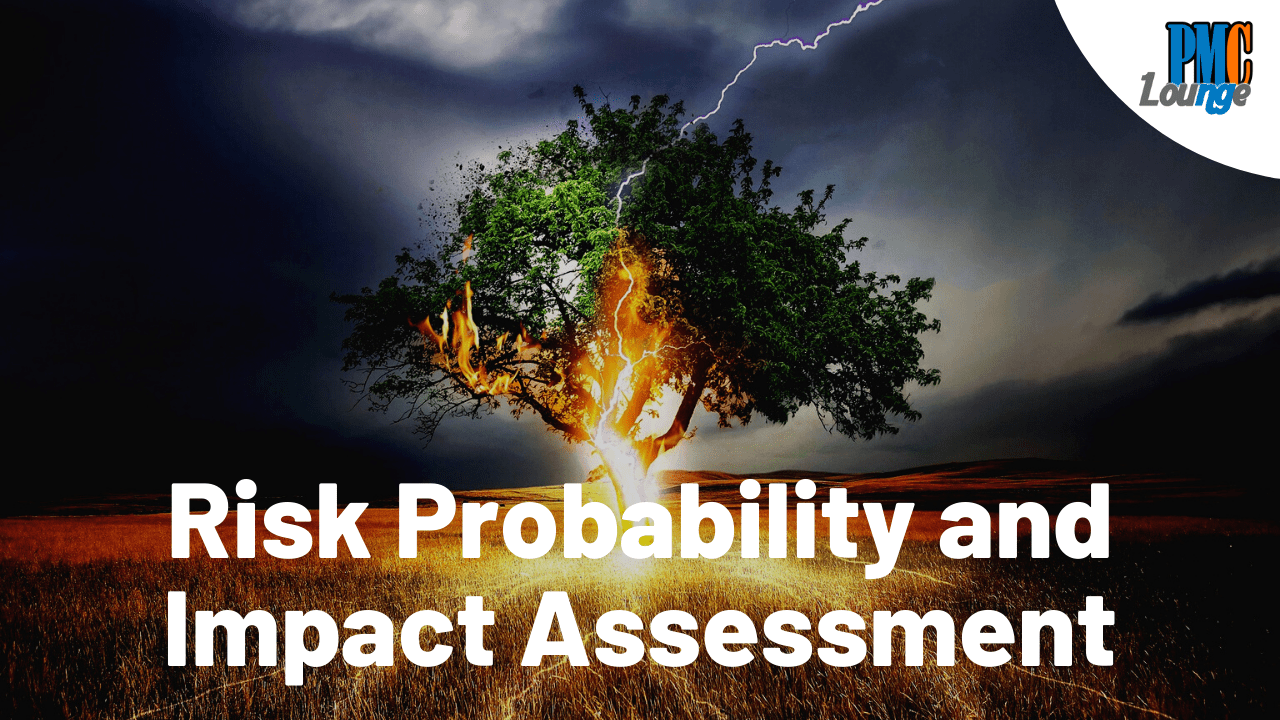# Risk Probability and Impact AssessmentHere’s a question, how do you determine if a risk is big or small? Also, how do you figure out how likely the risk is going to occur or materialize?

There is a section in the Risk Management Plan for this, it is known as the Definitions of Probability and Impact. In order to handle risks,

1. You need to figure out how likely is it that the risk is going to materialize. You do this by assigning a probability value based on the likelihood of the risk’s occurrence
2. Once you have the probability figured out, you then work towards estimating the cost or the impact of the risk if it does materialize

Using these values, you will be able to interpret which risks need a pretty solid mitigation plan while which ones can be monitored as the project progresses

## Example of Definition of Impact Assessment

Check more articles on Risk Management